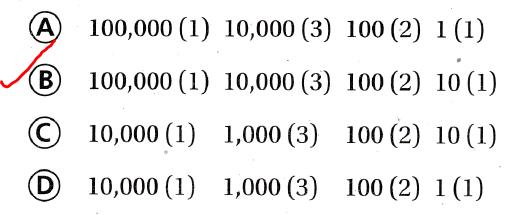Refer to our Texas Go Math Grade 4 Answer Key Pdf to score good marks in the exams. Test yourself by practicing the problems from Texas Go Math Grade 4 Lesson 1.2 Answer Key Read and Write Numbers.

Try This! Use place value to read and write numbers.

Standard Form: 475,856,144
Word form: four hundred seventy-five ___________, eight hundred fifty-six ___________, one hundred forty-four
The given number is: 475,856,144
Now,
We know that,
“Word form” is writing the numerical/number as you would say it in words
Hence, from the above,
We can conclude that
The representation of the given number in the Word form is:
four hundred seventy-five million eight hundred fifty-six thousand one hundred forty-four

Expanded Form: ___________ + 70,000,000 + 5,000,000 + ___________ + ___________+ 6,000 + ___________ + 40 + 4
The given number is: 475,856,144
Now,
We know that,
The “Expanded form” is a way to write a number by adding the value of its digits
Hence, from the above,
We can conclude that
The representation of the given number in the expanded form is:
40,000,000 + 70,000,000 + 5,000,000 + 8,00,000 + 50,000 + 6,000 + 100 + 40 + 4

Share and Grow

Question 1.
How can you use place value and period names to read and write 12,324,904 in word form?
The given number is: 12.324,904
Now,
The representation of the given number in the place-value chart is: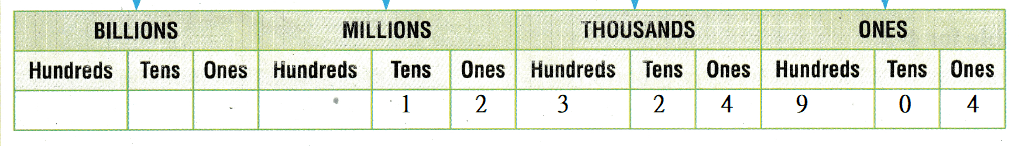Now,
From the above place-value chart,
We can observe that
The number can be represented in the following periods: Millions, Thousands, Ones
Hence, from the above,
We can conclude that
The representation of 12,324,904 in the word form is:
Twelve million three hundred twenty-four thousand nine hundred four

Read and write the number in two other forms.

Question 2.
four hundred eight thousand, seventeen
The given word form is:
Four hundred eight thousand, seventeen
Now,
We know that,
“Expanded form” means writing the number so you show the value of each digit.
“Word form” means writing the number with words instead of numerals.
Hence, from the above,
We can conclude that
The representation of the given word form in Standard form is: 408,017
The representtaion of the given word form in Expanded form is:
400,000 + 0 + 8,000 + 0 + 10 + 7

Question 3.
65,058
The given standard form is: 65,058
Now,
We know that,
“Expanded form” means writing the number so you show the value of each digit.
“Word form” means writing the number with words instead of numerals.
Hence, from the above,
We can conclude that
The representation of the given standard form in Word form is:
Sixty-five thousand and fifty-eight
The representtaion of the given Standard form in Expanded form is:
60,000 + 5,000 + 0 + 50 + 8

Problem Solving

Use the table 4-5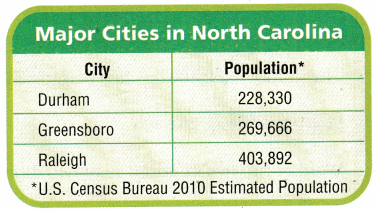Question 4.
Use Diagrams Which city has a population of two hundred sixty-nine thousand, six hundred sixty-six?
The given table is:Now,
The given word form is:
Two hundred sixty-nine thousand, six hundred sixty-six
Now,
We know that,
“Expanded form” means writing the number so you show the value of each digit.
“Word form” means writing the number with words instead of numerals.
So,
The representation of the given word form in the Standard form is: 269,666
Hence, from the above,
We can conclude that
The city that has a population of two hundred sixty-nine thousand, six hundred sixty-six is: Greensboro

Question 5.
Write the population of Raleigh in expanded form and word form.
The given table is:Now,
From the given table,
We can observe that
The population of Raleigh is: 403,892
Now,
We know that,
“Expanded form” means writing the number so you show the value of each digit.
“Word form” means writing the number with words instead of numerals.
Hence, from the above,
We can conclude that
The representation of the population of Raleigh in the Word form is:
Four hundred and three thousand, Eight hundred and Ninety-two
The representation of the population of raleigh in the Expanded form is:
400,000 + 0 + 3,000 + 800 + 90 + 2

Question 6.HOT Multi-Step: What’s the Error?
Sophia said that the expanded form for 605,970 is 600,000 + 50,000 + 900 + 70. Describe Sophia’s error and give the correct answer.
It is given that
Sophia said that the expanded form for 605,970 is 600,000 + 50,000 + 900 + 70
Now,
The given number is: 605,970
Now,
The representation of the number in the place-value chart is: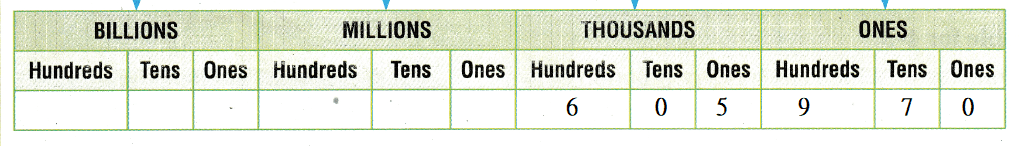Now,
From the above place-value chart,
We can observe that
The ten thousand’s place for the given number is 0
So,
The representtaion of Sophia’s number in the Expanded form is:
600,000 + 0 + 5,000 + 900 + 70 + 0
Hence, from the above,
We can conclude that
Sophia’s error is:
The ten thousand’s place for the given number is 0
The correct representation of Sophia’s number in the expanded form is:
600,000 + 0 + 5,000 + 900 + 70 + 0

Unlock the problems

Question 7.
H.O.T.
Mark tossed six balls while playing a number game. Three balls landed in one section, and three balls landed in another section. His score is greater than one hundred thousand. What could his score be?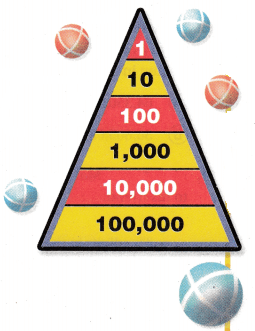a. What do you know?
The given information is:
Mark tossed six balls while playing a number game. Three balls landed in one section, and three balls landed in another section. His score is greater than one hundred thousand
Now,
We know that,
The given points table is:b. How can you use what you know about place value to find what Mark’s score could be?
The given points table is:Now,
We know that,
The place values increase from left to right in the place value chart
So,
By using the above points table,
Represent the points that the balls are tossed in the place-value chart and add them and it will be Mark’s score

c. Draw a diagram to show one way to solve the problem.
The diagram that shows the way to solve the given problem is: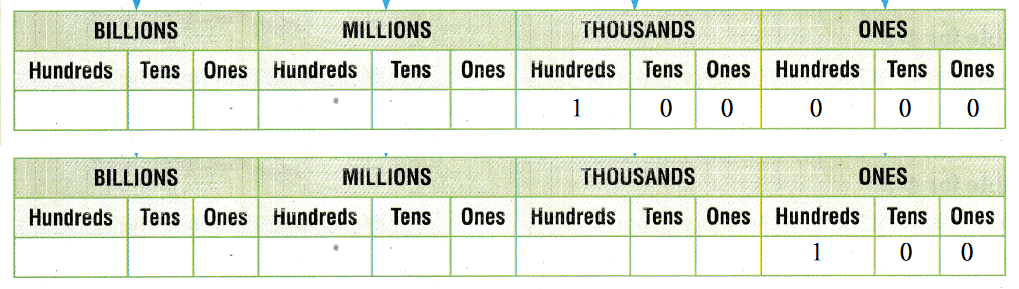d. Complete the sentences.
Three balls could have landed in the “Thousands” section.
Three balls could have landed in the One’s section.
So,
Mark’s score = 100,000 + 100
= 100,100
Hence, from the above,
We can conclude that
Mark’s score could be: 100,100

Question 8.
There are 2,750 sheep on a farm. Write the number of sheep in word form and expanded form.
It is given that
There are 2,750 sheep on a farm
Now,
We know that,
“Expanded form” means writing the number so you show the value of each digit.
“Word form” means writing the number with words instead of numerals.
Hence, from the above,
We can conclude that
The representation of 2,750 in the Word form is:
Two thousand and Seven hundred and fifty
The representation of 2,750 in the Expanded form is:
2,000 + 700 + 50 + 0

Question 9.
What is twenty-seven million, four hundred eight thousand, nine hundred forty-five written in standard form?
The given word form is:
Twenty-seven million, four hundred eight thousand, nine hundred forty-five
Now,
We know that,
“Expanded form” means writing the number so you show the value of each digit.
“Word form” means writing the number with words instead of numerals.
Hence, from the above,
We can conclude that
The representation of the given Word form in the Standard form is: 27,408,945

Question 10.
Jen wrote sixty-four million, three hundred five thousand, twenty-one miles on the board. Which is this number written in standard form?
(A) 64,350,210 miles
(B) 30,605,021 miles
(C) 64,021,305 miles
(D) 64,305,021 miles
It is given that
Jen wrote sixty-four million, three hundred five thousand, twenty-one miles on the board
Now,
We know that,
“Expanded form” means writing the number so you show the value of each digit.
“Word form” means writing the number with words instead of numerals.
So,
The representation of the given Word form in the Standard form is: 64,305,021
Hence, from the above,
We can conclude that
The representation of Jen’s number in the standard form is:Question 11.
Multi-Step Mark used these clues to describe a number to his friend.

• The digits in the one’s period are 682.
• The word form of the thousand periods is seven hundred thirty thousand.
• The digits in the hundred million and one million places are the same.

Which number could Mark have described?
(A) 682,730,151
(B) 151,703,682
(C) 730,151,682
(D) 151,730,682
The clues used by Mark are:
a. The digits in the one’s period are 682.
b. The word form of the thousand periods is seven hundred thirty thousand.
c. The digits in the hundred million and one million places are the same.
Now,
The representation of Mark’s clues in the place-value chart is:Hence, from the above,
We can conclude that
The number mark could have described is: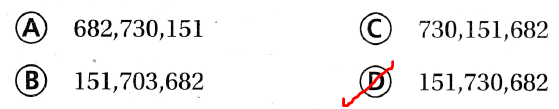Question 12.
Which shows 5,610,050,004 written in expanded form?
(A) 50,000,000 + 6,000,000 + 100,000 + 50,000 + 4
(B) 600,000,000 + 20,000,000 + 4,000,000 + 5,000 + 400 + 5
(C) 5,000,000,000 + 600,000,000 + 10,000,000 + 50,000 + 4
(D) 5,000,000 + 600,000 + 10,000 + 5,000 + 0 + 4
The given number is: 5,610,050,004
Now,
We know that,
“Expanded form” means writing the number so you show the value of each digit.
Hence, from the above,
We can conclude that
The representation of the given number in the Expanded form is:Texas Test Prep

Question 13.
The new football stadium was filled to capacity with 105,840 fans. What is the value of the digit 5 in 105,840?
(A) 500
(B) 5,000
(C) 50,000
(D) 500,000
It is given that
The new football stadium was filled to capacity with 105,840 fans
Now,
The given number is: 105,840
So,
The representation of the given number in the place-value chart is:Hence, from the above,
We can conclude that
The value of the digit 5 in the given number is: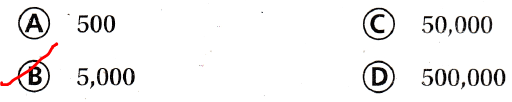### Texas Go Math Grade 4 Lesson 1.2 Homework and Practice Answer Key

Use the table for 1-4.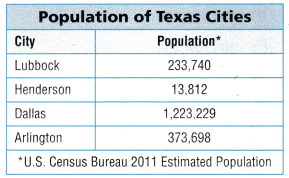Question 1.
Write the population of Lubbock in word form.
The given table is:Now,
From the given table,
We can observe that
The population of Lubbock is: 233,740
Now,
We know that,
“Expanded form” means writing the number so you show the value of each digit.
“Word form” means writing the number with words instead of numerals.
Hence, from the above,
We can conclude that
The representation of the population of Lubbock in the Word form is:
Two hundred and thirty-three thousand, Seven hundred and forty

Question 2.
What is the expanded form for the population of Arlington?
The given table is:Now,
From the above,
We can observe that
The population of Arlington is: 373,698
Now,
We know that,
“Expanded form” means writing the number so you show the value of each digit.
“Word form” means writing the number with words instead of numerals.
Hence, from the above,
We can conclude that
The representation of the population of Arlington in the Expanded form is:
300,000 + 70,000 + 3,000 + 600 + 90 + 8

Problem Solving

Question 3.
All four cities have the same digit in one place. What is the place? Write the value of the digit.
The given table is:Now,
From the given table,
We can observe that
The thousand’s place has the same digit in the populations of all the four cities
Hence, from the above,
We can conclude that
All four cities have the same digit in one place. That place is: Thousand
The value of the digit is: 3,000

Question 4.
Which city has a population that is about 100 times the population of Henderson? write the population in word form.
The given table is:Now,
From the above table,
We can observe that
The population of Henderson is: 13,812
Now,
The 100 times as much as the population as Henderson = 100 × 13,812
= 1,381,200
Now,
From the given table,
We can observe that
The population of Dallas is very near to Henderson
Hence, from the above,
We can conclude that
The city has a population that is about 100 times the population of Henderson is: Dallas

Question 5.
Which shows three hundred five million, two hundred eighty thousand, four hundred ninety-six written in standard form?
(A) 300,528,496
(B) 350,208,469
(C) 305,028,496
(D) 305,280,496
The given word form is:
Three hundred five million, two hundred eighty thousand, four hundred ninety-six
Now,
We know that,
“Expanded form” means writing the number so you show the value of each digit.
“Word form” means writing the number with words instead of numerals.
Hence, from the above,
We can conclude that
The representation of the given Word form in the standard form is: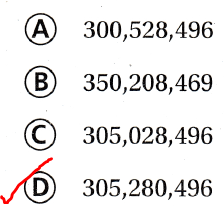Question 6.
During the week of the county fair, 15,609 entry tickets were sold. Which shows 15,609 written in expanded form?
(A) 10,000 + 5,000 + 600 + 9
(B) 10,000 + 5,000 + 600 + 90
(C) 1,000 + 500 + 60 + 9
(D) 1,000 + 600 + 9
It is given that
During the week of the county fair, 15,609 entry tickets were sold
Now,
We know that,
“Expanded form” means writing the number so you show the value of each digit.
“Word form” means writing the number with words instead of numerals.
Hence, from the above,
We can conclude that
The representation of the given number in the Expanded form is:Question 7.
There were 94,172 people at a football game. Which digit is in the thousands’ place in this number?
(A) 9
(B) 1
(C) 4
(D) 7
It is given that
There were 94,172 people at a football game
Now,
The given number is: 94,172
Now,
The representation of the given number in the place-value chart is:Hence, from the above,
We can conclude that
The digit in the thousand’s place in the given number is:Question 8.
Richard got 5,263,148 hits when he did an Internet search. What is the value of the digit 6 in this number?
(A) 60,000
(B) 600,000
(C) 6,000
(D) 6,000,000
It is given that
Richard got 5,263,148 hits when he did an Internet search
Now,
The given digit is: 5,263,148
Now,
The representation of the given digit in the place-value chart is: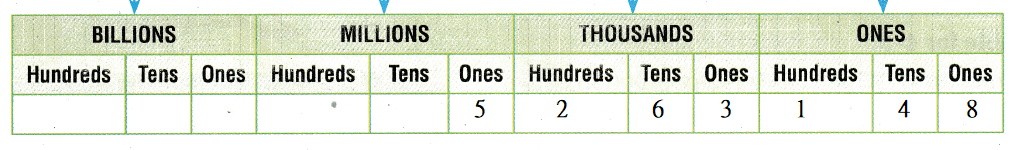Hence, from the above,
We can conclude that
The value of digit 6 in the given number is: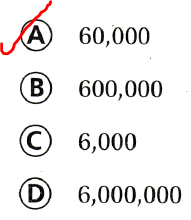Question 9.
Multi-Step:
Mason tossed 6 balls onto this number board. Two landed on 10,000. Two others landed on 100,000. One ball landed on 1. One ball landed on 100. What is Mason’s score?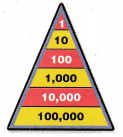A. 220,101
B. 22,101
C. 2,121
D. 210,201
It is given that
Mason tossed 6 balls onto this number board. Two landed on 10,000. Two others landed on 100,000. One ball landed on 1. One ball landed on 100
So,
According to the given information,
The total score of Mason = (2 × 10,000) + (2 × 100,000) + (1 × 1) + (1 × 100)
= 20,000 + 200,000 + 1 + 100
= 220,000 + 101
= 220,101
Hence, from the above,
We can conclude that
The total score of Mason is:Question 10.
Multi-Step:
Katy tossed 7 balls onto this number board. Her score was 130,210. Which correctly lists the sections where the balls landed?
A. 100,000(1) 10,000(3) 100(2) 1 (1)
B. 100,000(1) 10,000(3) 100(2) 10(1)
C. 10,000(1) 1,000(3) 100(2) 10(1)
D. 10,000(1) 1,000(3) 100(2) 1 (1)